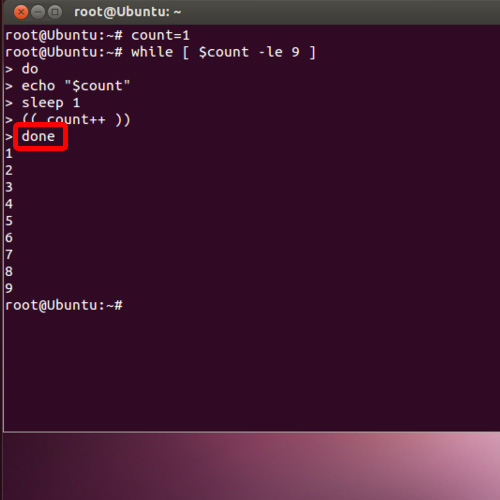## How to Write Bash While-Loops

In this tutorial we will show you how to write bash while loops. Linux can be used to enter commands where we can specify the number of times a loop will run. This can be done by writing a script for a bash while loop.
To understand how to create a while-loop in bash, follow the steps given below.

Step 1 – Define the starting point

First of all, we will define from where the count will start from.
count=1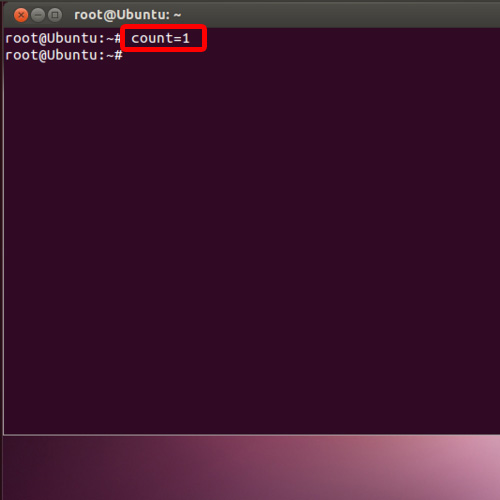Step 2 – Define the number of times the loop with run

Next, we will define how many times the loop will run. Over here, we have defined it as “less than equal to” 9.
while [ \$count –le 9 ]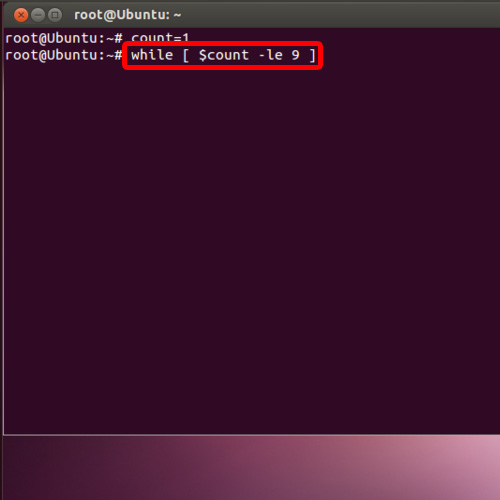Step 3 – Enter the “do” command

Now we start with the loop structure and for that we will type “do” over here.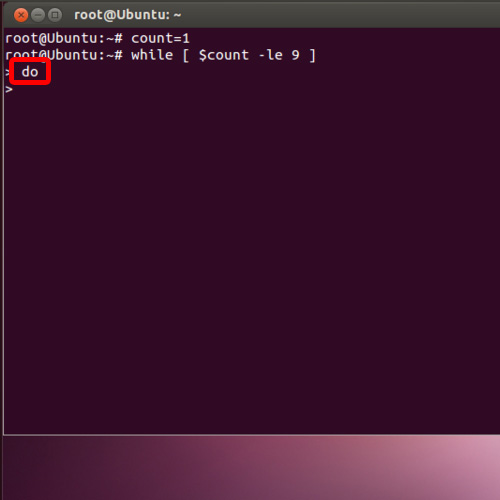Step 4 – Print the output

Next we will print the output using the echo command over here.
echo “\$count”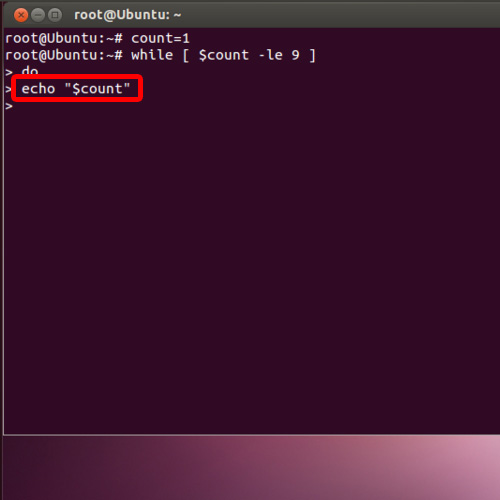Step 5 – Use the sleep command

Since we don’t want the output be displayed all at once, we have used the sleep command over here, so that there is an interval of one second after each result is displayed.
sleep 1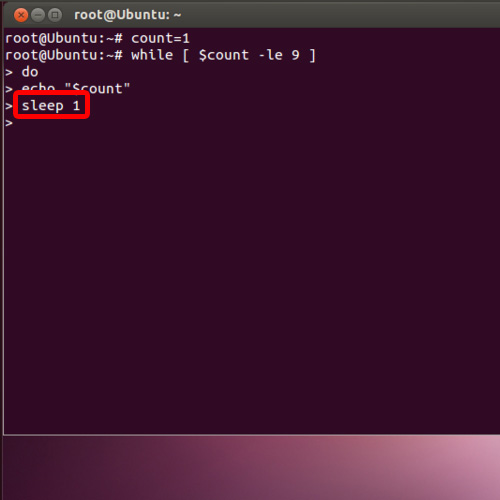Step 6 – Specify output to be increased by 1

Next, we will specify that after each result is displayed, the output should be incremented by 1 in this specific bash while loop.

(( count++ ))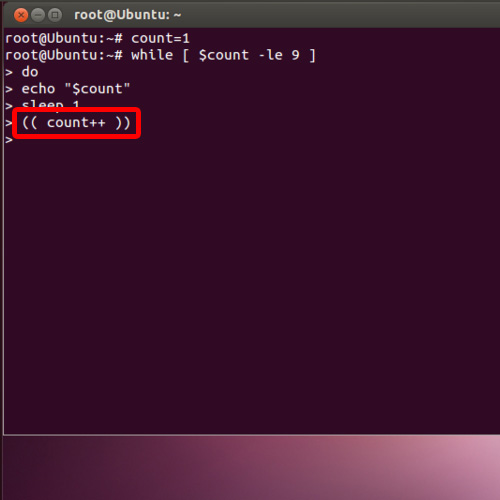Step 7 – Enter the “Done” command

Now let’s write “Done” over here and all the numbers will be automatically be displayed, after an interval of one second.
And that’s it, by following the above steps and even changing a few numbers you can create your own bash while loops.

done